## Abstract

In this article, the ${\textstyle exp(-\Phi (\xi ))}$-expansion method is modified for (3+1)-dimensional space–time coordinate system and successfully implemented to construct the new exact traveling wave solutions of the (3+1)-dimensional coupled Klein–Gordon–Zakharov equation. The solutions of this equation are expressed in terms of hyperbolic, trigonometric, exponential and rational functions. The results illustrate its effectiveness for solving nonlinear coupled partial differential equations arises in mathematical physics and engineering. The annihilation phenomena of the wave propagation in the ${\textstyle x}$${\textstyle y}$ plane are also investigated. Furthermore, the three-dimensional surface plots due to the obtained solutions are also given to make the dynamics of the equation visible.

## Keywords

The nonlinear coupled Klein–Gordon–Zakharov equation; Traveling wave solutions; Solitary wave solutions; The exp(-Φ(ξ))exp(-Φ(ξ))-expansion method

## 1. Introduction

It is well known that partial differential equations (PDEs) are special kind of nonlinear evolution equations. Already a large number of authors ,  and  have derived the nonlinear PDEs considering different theoretical model equations. Many issues of physical phenomena in nature may be described by solving the nonlinear PDEs. The explicit solutions of these equations play a vital role to study such physical phenomena in mathematical physics. Thus, the nonlinear equations may become most exciting and enormously active research areas for mathematicians, physicists and engineers. On the other hand, the traveling wave solutions play an essential role to study the models arising from various natural and complex phenomena in the field of applied sciences and engineering. For instance, the wave propagation phenomena were observed in optical fibers, elastic media, quantum mechanics, fluids dynamics, chemical physics, solid mechanics, biophysics, Higgs mechanism, quantum field theory, plasma physics and so on. To obtain the exact solutions of the nonlinear PDEs, the initial dominant mathematical tool was the inverse scattering method . Some of other most efficient methods for finding analytical solutions of the nonlinear PDEs are the three-wave method , the improved F-expansion method , the generalized method , the Weiss–Tabor–Carnevale method , the tanh-function method , the extended tanh-method  and , the modified extended tanh-function method  and , the Jacobi elliptic function expansion method , the extended Jacobi elliptic function expansion rational method , the Exp-function method ,  and , the truncated Painleve expansion method , the homogeneous balance method ,  and  and so on. Recently, the ${\textstyle exp(-\Phi (\xi ))}$-expansion method , , ,  and  has also been used to find the exact traveling wave solutions of single nonlinear PDEs. Moreover, many researchers , , ,  and  have investigated the exact solutions of the coupled nonlinear PDEs, that is, the Maccari system, Higgs field equation, (1+1)-dimensional nonlinear coupled Klein–Gordon–Zakharov equation, etc. employing the Exp-function method , truncated expansion method , first integral method , and conservative difference methods .

Many types of coupled Klein–Gordon–Zakharov equation ,  and  have obtained to study the nonlinear propagation of physical phenomena in physics, especially in plasma physics and fluid dynamics. In order to get the plane wave solutions for the coupled nonlinear evolution equations in three dimensional space and time, we have modified the ${\textstyle exp(-\Phi (\xi ))}$-expansion method for solving (3+1)-dimensional coupled nonlinear evolution equations. The aim of the present work was to find the exact traveling wave solutions of (3+1)-dimensional nonlinear coupled Klein–Gordon–Zakharov equation. Thus, the paper is arranged as follows: Modification of the ${\textstyle exp(-\Phi (\xi ))}$ expansion method is given in Section 2. The traveling wave solutions of (3+1)-dimensional coupled Klein–Gordon–Zakharov equation are depicted in Section 3. The physical significances along with relevant discussion are mentioned in Section 4. Finally, the conclusion is drawn in Section 5.

## 2. Description of the modified exp(−Φ(ξ))-expansion method

The general form of the nonlinear PDE can be written as follows:

 $F(u{\mbox{,}}u_{t}{\mbox{,}}u_{x}{\mbox{,}}u_{y}{\mbox{,}}u_{z}{\mbox{,}}u_{xx}{\mbox{,}}u_{yy}{\mbox{,}}u_{zz}{\mbox{,}}u_{tt}{\mbox{,}}u_{tx}{\mbox{,}}\ldots )=$$0{\mbox{,}}$
(1)

where ${\textstyle u=u({\overset {\rightarrow }{r}}{\mbox{,}}t)}$ is an unknown function of ${\textstyle x{\mbox{,}}y{\mbox{,}}z}$ and ${\textstyle t}$, ${\textstyle F}$ is a polynomial in ${\textstyle u({\overset {\rightarrow }{r}}{\mbox{,}}t)}$ and its derivative in which higher order derivatives and nonlinear terms involved and the subscripts stand for the partial derivatives.

Now, combining the real variables ${\textstyle x{\mbox{,}}y{\mbox{,}}z}$ and t   by a compound variable ${\textstyle \xi }$ is as follows:

 $u({\overset {\rightarrow }{r}}{\mbox{,}}t)=u(\xi ){\mbox{,}}\quad \xi =$${\overset {\rightarrow }{P}}\cdot {\overset {\rightarrow }{r}}\pm \omega t{\mbox{,}}$
(2)

Here ${\textstyle {\overset {\rightarrow }{P}}=p{\hat {i}}+q{\hat {j}}+r{\hat {k}}}$, ${\textstyle {\overset {\rightarrow }{r}}=x{\hat {i}}+y{\hat {j}}+z{\hat {k}}}$, where ${\textstyle p{\mbox{,}}q{\mbox{,}}r}$ are the constant magnitude along the axes of ${\textstyle x{\mbox{,}}y{\mbox{,}}z}$ respectively and ${\textstyle \omega }$ is the speed of the traveling wave. Using the traveling wave variables transformation (2), Eq. (1) can be reduced to an ordinary differential equation (ODE) for ${\textstyle u=u(\xi )}$:

 $R(u{\mbox{,}}u^{'}{\mbox{,}}u^{''}{\mbox{,}}u^{'''}{\mbox{,}}\ldots )=$$0{\mbox{,}}$
(3)

where ${\textstyle R}$ is a polynomial of u   and its derivatives and primes indicate the ordinary derivatives with respect to ${\textstyle \xi }$.

The traveling wave solution of Eq. (3) can be expressed as follows:

 $u(\xi )=\sum _{i=0}^{N}A_{i}{\left(exp(-\Phi (\xi ))\right)}^{i}{\mbox{,}}$
(4)

where ${\textstyle A_{i}\quad (0\leqslant i\leqslant N)}$ are constants to be evaluated later, such that ${\textstyle A_{N}\quad \not =\quad 0}$ and ${\textstyle \Phi =\Phi (\xi )}$ satisfy the following ODE:

 ${\Phi }^{'}(\xi )=exp(-\Phi (\xi ))+\mu exp(\Phi (\xi ))+$$\lambda {\mbox{,}}$
(5)

where ${\textstyle \lambda }$ and ${\textstyle \mu }$ are arbitrary constants.

It is noted that Eq. (5) gives the following general solutions:

• Type 1: When ${\textstyle \mu \quad \not =\quad 0{\mbox{,}}\quad \Theta ={\lambda }^{2}-}$$4\mu >0$,
 $\Phi (\xi )=ln\left({\frac {-{\sqrt {\Theta }}tanh\left\{0.5{\sqrt {\Theta }}(\xi +E)\right\}-\lambda }{2\mu }}\right)$
(6)
• Type 2: When ${\textstyle \mu \quad \not =\quad 0{\mbox{,}}\quad \Theta ={\lambda }^{2}-}$$4\mu <0$,
 $\Phi (\xi )=ln\left({\frac {{\sqrt {-\Theta }}tan\left\{0.5{\sqrt {-\Theta }}(\xi +E)\right\}-\lambda }{2\mu }}\right)$
(7)
• Type 3: When ${\textstyle \mu =0{\mbox{,}}\quad \lambda \quad \not =\quad 0}$, and ${\textstyle \Theta ={\lambda }^{2}-4\mu >0}$,
 $\Phi (\xi )=-ln\left({\frac {\lambda }{exp(\lambda (\xi +E))-1}}\right)$
(8)
• Type 4: When ${\textstyle \mu \quad \not =\quad 0{\mbox{,}}\quad \lambda \quad \not =}$$\quad 0$, and ${\textstyle \Theta ={\lambda }^{2}-4\mu =0}$,
 $\Phi (\xi )=ln\left(-{\frac {2(\lambda (\xi +E)+2)}{{\lambda }^{2}(\xi +E)}}\right)$
(9)
• Type 5: When ${\textstyle \mu =0{\mbox{,}}\quad \lambda =0}$, and ${\textstyle \Theta ={\lambda }^{2}-4\mu =0}$,
 $\Phi (\xi )=ln\left(\xi +E\right)$
(10)

where E is the integration constant.

The value of the positive integer N can be determined by balancing the higher order derivative linear terms with the nonlinear terms of the highest order that appeared in Eq. (3). If the degree of ${\textstyle u(\xi )}$ is ${\textstyle D[u(\xi )]=N}$, then the degree of the other expressions can be evaluated by the following formulae:

 $D\left[{\frac {d^{p}u(\xi )}{d{\xi }^{p}}}\right]=N+p{\mbox{,}}\quad D\left[u^{p}{\left({\frac {d^{q}u(\xi )}{d{\xi }^{q}}}\right)}^{s}\right]=$$Np+s(N+q){\mbox{.}}$

By substituting Eq. (4) into Eq. (3) and using (5) rapidly, one can obtain a system of algebraic equations by equating all the coefficients of ${\textstyle exp(-\Phi (\xi ))}$ to zero. The obtaining system can be solved to find the value of ${\textstyle A_{1}{\mbox{,}}A_{2}\ldots {\mbox{,}}\omega {\mbox{,}}p{\mbox{,}}q{\mbox{,}}r}$ with the help of symbolic computation software such as Maple. Substituting the values of ${\textstyle A_{1}{\mbox{,}}A_{2}\ldots {\mbox{,}}\omega {\mbox{,}}p{\mbox{,}}q{\mbox{,}}r}$ into Eq. (4) along with general solutions of Eq. (5) completes the determination of the solution of Eq. (1).

Advantages: The advantage of the proposed method over other existing method is that it is provided the new exact traveling wave solutions along with additional free parameters. The exact solutions have its great importance to reveal the inner mechanism of the natural physical phenomena. The obtaining solutions of nonlinear evolution equations may be helpful for the numerical solvers to compare the correctness of their results and stability analysis. Various types of traveling wave solutions are obtained when the related physical parameters received their particular values. Algebraic manipulation of the proposed scheme is much easier rather than the other methods with the help of symbolic computation, such as, Maple. The effectiveness of this method is provided in the next section.

## 3. Application of the method

This section presents the application of the modified ${\textstyle exp(-\Phi (\xi ))}$-expansion method for finding the exact traveling wave solutions of the (3+1)-dimensional coupled Klein–Gordon Zakharov equation .

Let us consider the coupled Klein–Gordon–Zakharov equation

 ${\begin{array}{l}u_{tt}-c_{0}^{2}{\nabla }^{2}u+f_{0}^{2}u+\delta uv=0\\v_{tt}-c_{0}^{2}{\nabla }^{2}v-\beta {\nabla }^{2}\left|u\right|^{2}=0\end{array}}{\mbox{.}}$
(11)

Here ${\textstyle u({\overset {\rightarrow }{r}}{\mbox{,}}t)}$ is a complex function describing the fast timescale component of electric field raised by electrons. ${\textstyle v({\overset {\rightarrow }{r}}{\mbox{,}}t)}$ is real function indicating the deviation of ion density form its equilibrium. The system of Eqs. (11) described the interaction of the Langmuir wave and the ion acoustic wave in high frequency plasma in plasma physics (more details in Ref. ).

To find out the wave packet solution, one can introduce the following transformation:

 $u({\overset {\rightarrow }{r}}{\mbox{,}}t)=exp\left\{i\left({\overset {\rightarrow }{k}}\cdot {\overset {\rightarrow }{r}}-\right.\right.$$\left.\left.\Omega t\right)\right\}U(\xi ){\mbox{,}}\quad v({\overset {\rightarrow }{r}}{\mbox{,}}t)=$$V(\xi ){\mbox{,}}$
(12)

with ${\textstyle {\overset {\rightarrow }{k}}\cdot {\overset {\rightarrow }{r}}=}$$k_{1}x+k_{2}y+k_{3}z$, ${\textstyle \xi ={\overset {\rightarrow }{P}}\cdot {\overset {\rightarrow }{r}}-}$$\omega t=px+qy+rz-\omega t$, where ${\textstyle k_{1}{\mbox{,}}k_{2}{\mbox{,}}k_{3}}$ are the constant magnitudes along the axes of ${\textstyle x{\mbox{,}}y{\mbox{,}}z}$ respectively. By using Eq. (12) into Eq. (11), one obtains the following coupled nonlinear ordinary differential equations:

 ${\begin{array}{l}\left({\omega }^{2}-c_{0}^{2}P^{2}\right)U^{''}+2i\left\{\omega \Omega -c_{0}^{2}\left(k_{1}p+k_{2}q+k_{3}r\right)\right\}U^{'}\\\quad -\left({\omega }^{2}-c_{0}^{2}K^{2}-f_{0}^{2}\right)U+\delta UV=0\\\left({\omega }^{2}-c_{0}^{2}P^{2}\right)V^{''}-\beta P^{2}{\left(U^{2}\right)}^{''}=0\end{array}}{\mbox{,}}$
(13)

where ${\textstyle K^{2}=k_{1}^{2}+k_{2}^{2}+k_{3}^{2}}$, ${\textstyle P^{2}=p^{2}+q^{2}+r^{2}}$ and primes denote the differentiation with respect to ${\textstyle \xi }$.

If we set ${\textstyle \omega ={\frac {c_{0}^{2}}{\Omega }}{\overset {\rightarrow }{k}}\cdot {\overset {\rightarrow }{P}}}$, then Eq. (13) becomes

 ${\begin{array}{l}\left({\omega }^{2}-c_{0}^{2}P^{2}\right)U^{''}-\left({\omega }^{2}-c_{0}^{2}K^{2}-f_{0}^{2}\right)U+\delta UV=0\\\left({\omega }^{2}-c_{0}^{2}P^{2}\right)V^{''}-\beta K^{2}{\left(U^{2}\right)}^{''}=0\end{array}}{\mbox{.}}$
(14)

Integrating the second equation of (14) twice with respect to ${\textstyle \xi }$, we obtain

 $V={\frac {C}{\left({\omega }^{2}-c_{0}^{2}P^{2}\right)}}+{\frac {\beta K^{2}}{\left({\omega }^{2}-c_{0}^{2}P^{2}\right)}}U^{2}$
(15)

where C is the integrating constant. By using (15) into the first equation of (14), we obtain

 ${\left({\omega }^{2}-c_{0}^{2}P^{2}\right)}^{2}U^{''}+\left\{\left({\omega }^{2}-\right.\right.$$\left.\left.c_{0}^{2}P^{2}\right)\left(-{\omega }^{2}+c_{0}^{2}K^{2}+\right.\right.$$\left.\left.f_{0}^{2}\right)+\delta C\right\}U+\delta \beta K^{2}U^{3}=$$0$
(16)

This is the elliptic-like equation with cubic nonlinearity. The pole of this equation is ${\textstyle N=1}$. Therefore, the ${\textstyle exp(-\Phi (\xi ))}$-expansion method allows us to use the solution in the following form:

 $U(\xi )=A_{0}+A_{1}exp(-\Phi (\xi )){\mbox{,}}\quad A_{1}\quad \not =$$\quad 0$
(17)

where ${\textstyle A_{0}}$ and ${\textstyle A_{1}}$ are unknown constants. By means of Eq. (17), Eq. (16) turns out into a polynomial of ${\textstyle exp(-\Phi (\xi ))}$ and equating the coefficient of ${\textstyle {\left(exp(-\Phi (\xi ))\right)}^{i}{\mbox{,}}\quad (i=}$$0{\mbox{,}}1{\mbox{,}}2{\mbox{,}}3)$ to zero, we obtain a system of algebraic equations as follows:

 $\delta \beta K^{2}A_{1}^{3}+2A_{1}c_{0}^{4}P^{4}+2{\omega }^{4}A_{1}-$$4{\omega }^{2}c_{0}^{2}P^{2}A_{1}=0{\mbox{.}}$
(18)

 $3A_{1}{\omega }^{4}\lambda -6A_{1}{\omega }^{2}c_{0}^{2}P^{2}\lambda +$$3\delta \beta K^{2}A_{0}A_{1}^{2}+3A_{1}c_{0}^{4}P^{4}\lambda =0$
(19)

 $2A_{1}{\omega }^{4}\mu -4A_{1}{\omega }^{2}c_{0}^{2}P^{2}\mu +3\delta \beta K^{2}A_{0}^{2}A_{1}+$$\delta {CA}_{1}+A_{1}{\omega }^{4}{\lambda }^{2}+{\omega }^{2}c_{0}^{2}P^{2}A_{1}-$$c_{0}^{2}P^{2}f_{0}^{2}A_{1}+A_{1}c_{0}^{4}P^{4}{\lambda }^{2}+{\omega }^{2}f_{0}^{2}A_{1}-$$2A_{1}{\omega }^{2}c_{0}^{2}P^{2}{\lambda }^{2}-c_{0}^{4}P^{2}K^{2}A_{1}+$${\omega }^{2}K^{2}+c_{0}^{2}A_{1}+2A_{1}c_{0}^{4}P^{4}\mu -{\omega }^{4}A_{1}=$$0$
(20)

 $-c_{0}^{4}P^{2}K^{2}A_{0}-2A_{1}{\omega }^{2}c_{0}^{2}P^{2}\lambda \mu +$$A_{1}{\omega }^{4}\lambda \mu +{\omega }^{2}c_{0}^{2}P^{2}A_{0}+$${\omega }^{2}K^{2}c_{0}^{2}A_{0}+\delta {CA}_{0}+\delta \beta K^{2}A_{0}^{3}-$$c_{0}^{2}P^{2}f_{0}^{2}A_{0}+{\omega }^{2}f_{0}^{2}A_{0}-{\omega }^{4}A_{0}+$$A_{1}c_{0}^{4}P^{4}\lambda \mu =0$
(21)

Solving the system algebraic Eqs. (18), (19), (20) and (21), we obtain the following set of solutions:

 $C=-{\frac {1}{2\delta }}4{\omega }^{4}\mu -8{\omega }^{2}c_{0}^{2}P^{2}\mu -$${\omega }^{4}{\lambda }^{2}\mu +2{\omega }^{2}c_{0}^{2}P^{2}-2c_{0}^{2}P^{2}f_{0}^{2}-$$c_{0}^{4}P^{4}{\lambda }^{2}+2{\omega }^{2}f_{0}^{2}+2{\omega }^{2}c_{0}^{2}P^{2}{\lambda }^{2}-$$2c_{0}^{4}P^{2}K^{2}+2{\omega }^{2}K^{2}c_{0}^{2}+4c_{0}^{4}P^{4}\mu -2{\omega }^{4}A_{0}=$${\frac {\pm i}{K{\sqrt {2\delta \beta }}}}\left(c_{0}^{2}P^{2}-\right.$$\left.{\omega }^{2}\right)\lambda {\mbox{,}}\quad A_{1}=$${\frac {\pm i}{K{\sqrt {2\delta \beta }}}}\left(c_{0}^{2}P^{2}-\right.$$\left.{\omega }^{2}\right){\mbox{.}}$
(22)

where ${\textstyle B_{0}{\mbox{,}}\lambda }$ and ${\textstyle \mu }$ are arbitrary constants.

Using (12), (15), (17) and (22), the traveling wave solutions of the coupled Klein–Gordon–Zakharov equation are obtained as follows:

When ${\textstyle \Theta ={\lambda }^{2}-4\mu >0{\mbox{,}}\quad \mu \quad \not =}$$\quad 0$, we have

 $u_{1}({\overset {\rightarrow }{r}}{\mbox{,}}t)=e^{i\left({\overset {\rightarrow }{k}}\cdot {\overset {\rightarrow }{r}}-\Omega t\right)}\left(c_{0}^{2}P^{2}-\right.$$\left.{\omega }^{2}\right){\frac {\pm i\lambda }{K{\sqrt {2\delta \beta }}}}-$${\frac {\pm i}{K{\sqrt {2\delta \beta }}}}\left({\frac {2\mu }{{\sqrt {\Theta }}tanh\left\{0.5{\sqrt {\Theta }}({\overset {\rightarrow }{p}}\cdot {\overset {\rightarrow }{r}}-\omega t+E)\right\}+\lambda }}\right)$
(23)
 $v_{1}({\overset {\rightarrow }{r}}{\mbox{,}}t)={\frac {C}{\left({\omega }^{2}-c_{0}^{2}P^{2}\right)}}-$$\beta K^{2}\left({\omega }^{2}-c_{0}^{2}P^{2}\right){\frac {\pm \lambda }{K{\sqrt {2\delta \beta }}}}\mp {\frac {1}{K{\sqrt {2\delta \beta }}}}{\left({\frac {2\mu }{{\sqrt {\Theta }}tanh\left\{0.5{\sqrt {\Theta }}({\overset {\rightarrow }{p}}\cdot {\overset {\rightarrow }{r}}-\omega t+E)\right\}+\lambda }}\right)}^{2}$
(24)

When ${\textstyle \Theta ={\lambda }^{2}-4\mu <0}$, we have

 $u_{2}({\overset {\rightarrow }{r}}{\mbox{,}}t)=e^{i\left({\overset {\rightarrow }{k}}\cdot {\overset {\rightarrow }{r}}-\Omega t\right)}\times \left(c_{0}^{2}P^{2}-\right.$$\left.{\omega }^{2}\right)\times \left\{{\frac {\pm i\lambda }{K{\sqrt {2\delta \beta }}}}+\right.$$\left.{\frac {\pm i}{K{\sqrt {2\delta \beta }}}}\left({\frac {2\mu }{{\sqrt {-\Theta }}tan\left\{0.5{\sqrt {-\Theta }}({\overset {\rightarrow }{p}}\cdot {\overset {\rightarrow }{r}}-\omega t+E)\right\}-\lambda }}\right)\right\}$
(25)
 $v_{2}({\overset {\rightarrow }{r}}{\mbox{,}}t)={\frac {C}{\left({\omega }^{2}-c_{0}^{2}P^{2}\right)}}-$$\beta K^{2}\left({\omega }^{2}-c_{0}^{2}P^{2}\right){\left\{{\frac {\pm \lambda }{K{\sqrt {2\delta \beta }}}}+{\frac {\pm 1}{K{\sqrt {2\delta \beta }}}}\left({\frac {2\mu }{{\sqrt {-\Theta }}tan\left\{0.5{\sqrt {-\Theta }}({\overset {\rightarrow }{p}}\cdot {\overset {\rightarrow }{r}}-\omega t+E)\right\}-\lambda }}\right)\right\}}^{2}$
(26)

When ${\textstyle {\lambda }^{2}-4\mu >0{\mbox{,}}\quad \mu =0}$, we have

 $u_{3}({\overset {\rightarrow }{r}}{\mbox{,}}t)=e^{i\left({\overset {\rightarrow }{k}}\cdot {\overset {\rightarrow }{r}}-\Omega t\right)}\left(c_{0}^{2}P^{2}-\right.$$\left.{\omega }^{2}\right)\times \left\{{\frac {\pm i\lambda }{K{\sqrt {2\delta \beta }}}}+\right.$$\left.{\frac {\pm i}{K{\sqrt {2\delta \beta }}}}\left({\frac {\lambda }{exp\left\{\lambda ({\overset {\rightarrow }{p}}\cdot {\overset {\rightarrow }{r}}-\omega t+E)\right\}-1}}\right)\right\}$
(27)
 $v_{3}({\overset {\rightarrow }{r}}{\mbox{,}}t)={\frac {C}{\left({\omega }^{2}-c_{0}^{2}P^{2}\right)}}-$$\beta K^{2}\left({\omega }^{2}-c_{0}^{2}P^{2}\right){\left\{{\frac {\pm \lambda }{K{\sqrt {2\delta \beta }}}}\pm {\frac {1}{K{\sqrt {2\delta \beta }}}}\left({\frac {\lambda }{exp\left\{\lambda ({\overset {\rightarrow }{p}}\cdot {\overset {\rightarrow }{r}}-\omega t+E)\right\}-1}}\right)\right\}}^{2}$
(28)

When ${\textstyle \mu \quad \not =\quad 0{\mbox{,}}\quad \lambda \quad \not =}$$\quad 0$, and ${\textstyle {\lambda }^{2}-4\mu =0}$, we have

 $u_{4}({\overset {\rightarrow }{r}}{\mbox{,}}t)=e^{i\left({\overset {\rightarrow }{k}}\cdot {\overset {\rightarrow }{r}}-\Omega t\right)}\times \left(c_{0}^{2}P^{2}-\right.$$\left.{\omega }^{2}\right)\times \left\{{\frac {\pm i\lambda }{K{\sqrt {2\delta \beta }}}}-\right.$$\left.{\frac {\pm i}{K{\sqrt {2\delta \beta }}}}\times \left({\frac {{\lambda }^{2}\left\{\lambda ({\overset {\rightarrow }{p}}\cdot {\overset {\rightarrow }{r}}+E)\right\}}{2\left\{\lambda ({\overset {\rightarrow }{p}}\cdot {\overset {\rightarrow }{r}}-\omega t+E)\right\}+4}}\right)\right\}$
(29)
 $v_{4}({\overset {\rightarrow }{r}}{\mbox{,}}t)={\frac {C}{\left({\omega }^{2}-c_{0}^{2}P^{2}\right)}}-$$\beta K^{2}\left({\omega }^{2}-c_{0}^{2}P^{2}\right)\left\{{\frac {\pm \lambda }{K{\sqrt {2\delta \beta }}}}-\right.$$\left.{\frac {\pm 1}{K{\sqrt {2\delta \beta }}}}\times \left({\frac {{\lambda }^{2}({\overset {\rightarrow }{p}}\cdot {\overset {\rightarrow }{r}}+E)}{2\left\{\lambda ({\overset {\rightarrow }{p}}\cdot {\overset {\rightarrow }{r}}-\omega t+E)\right\}+4}}\right)\right\}$
(30)

When ${\textstyle \mu =0{\mbox{,}}\quad \lambda =0}$, and ${\textstyle {\lambda }^{2}-4\mu =0}$, we have

 $u_{5}({\overset {\rightarrow }{r}}{\mbox{,}}t)=e^{i\left({\overset {\rightarrow }{k}}\cdot {\overset {\rightarrow }{r}}-\Omega t\right)}\times \left(c_{0}^{2}P^{2}-\right.$$\left.{\omega }^{2}\right)\times \left\{{\frac {\pm i\lambda }{K{\sqrt {2\delta \beta }}}}-\right.$$\left.{\frac {\pm i}{K{\sqrt {2\delta \beta }}}}\left({\frac {1}{{\overset {\rightarrow }{p}}\cdot {\overset {\rightarrow }{r}}-\omega t+E}}\right)\right\}$
(31)
 $v_{5}({\overset {\rightarrow }{r}}{\mbox{,}}t)={\frac {C}{\left({\omega }^{2}-c_{0}^{2}P^{2}\right)}}-$$\beta K^{2}\left({\omega }^{2}-c_{0}^{2}P^{2}\right){\left\{{\frac {\pm \lambda }{K{\sqrt {2\delta \beta }}}}-{\frac {\pm 1}{K{\sqrt {2\delta \beta }}}}\left({\frac {1}{{\overset {\rightarrow }{p}}\cdot {\overset {\rightarrow }{r}}-\omega t+E}}\right)\right\}}^{2}$
(32)

The above determined solutions may be useful for researchers to understand the interaction of the Langmuir wave and the ion acoustic wave in high frequency plasma in plasma physics. The physical significances of these solutions are also described in the next section.

## 4. Physical significance

This section presents the physical explanation of traveling wave solutions to the coupled Klein–Gordon–Zakharov equation in the ${\textstyle x}$${\textstyle y}$ plane for different values of time t  . The coupled Klein–Gordon–Zakharov equation are given various types of traveling wave propagation in the ${\textstyle x}$${\textstyle y}$ plane, such as, kink type, singular kink type, soliton type, cupson type and bell type solitary waves as well as periodic traveling waves. It is noted the delicate balance between linear and nonlinear effects on the solitons solution which are fully interacting with others and come back with the same speed and shape. That is, the amplitude, velocity and wave shape of solitons remain unchanged after nonlinear interaction and showing completely elastic collisions. However, some solutions of coupled Klein–Gordon–Zakharov equation in the ${\textstyle x}$${\textstyle y}$ plane for different values of time among solitonic excitations are not completely elastic, because their shapes are changed after their interactions. Thus, the traveling wave solutions are described physically with elastic property and presented graphically in Figure 1, Figure 2, Figure 3, Figure 4, Figure 5, Figure 6, Figure 7, Figure 8, Figure 9 and Figure 10.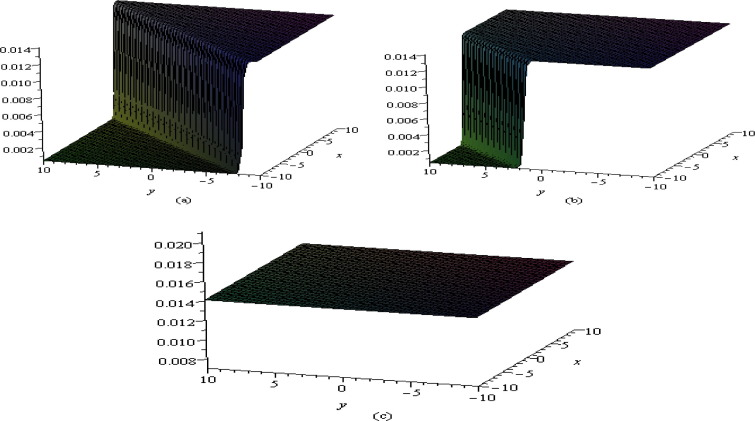Figure 1. Shape of ${\textstyle \vert u_{1}(x{\mbox{,}}y)\vert }$ in ${\textstyle x}$–${\textstyle y}$ plane at (a) ${\textstyle t=0}$, (b) ${\textstyle t=10}$ and (c) ${\textstyle t=30}$.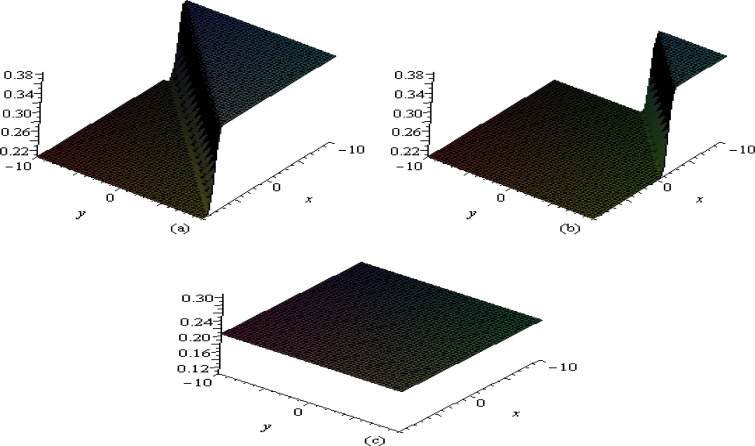Figure 2. Shape of ${\textstyle {\nu }_{1}}$ in ${\textstyle x}$–${\textstyle y}$ plane at (a) ${\textstyle t=0}$, (b) ${\textstyle t=10}$ and (c) ${\textstyle t=30}$.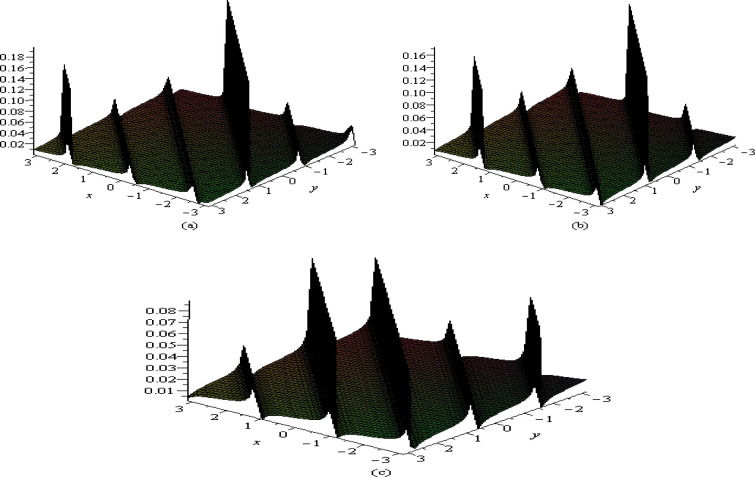Figure 3. Shape of ${\textstyle \vert u_{2}\vert }$ in ${\textstyle x}$–${\textstyle y}$ plane at (a) ${\textstyle t=0}$, (b) ${\textstyle t=10}$ and (c) ${\textstyle t=1000}$.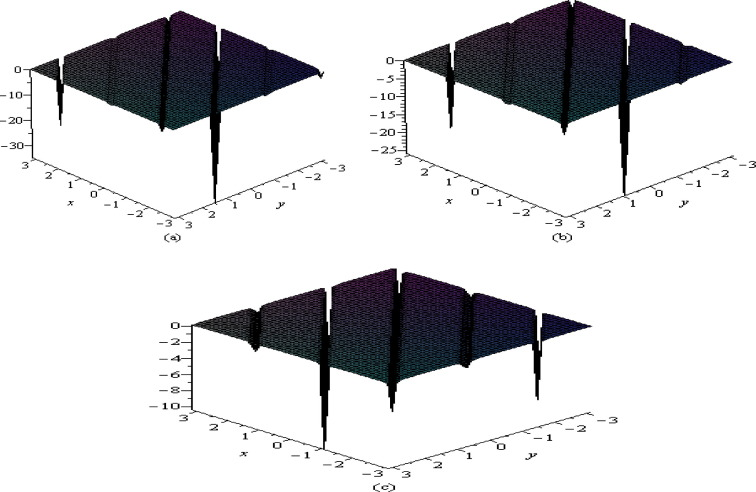Figure 4. Shape of ${\textstyle {\nu }_{2}}$ in ${\textstyle x}$–${\textstyle y}$ plane at (a) ${\textstyle t=0}$, (b) ${\textstyle t=10}$ and (c) ${\textstyle t=100}$.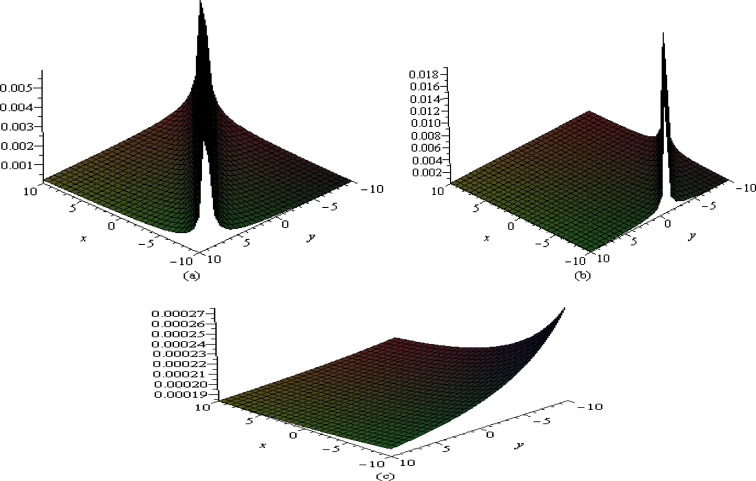Figure 5. Shape of ${\textstyle \vert u_{3}\vert }$ in ${\textstyle x}$–${\textstyle y}$ plane at (a) ${\textstyle t=0}$, (b) ${\textstyle t=10}$ and (c) ${\textstyle t=30}$.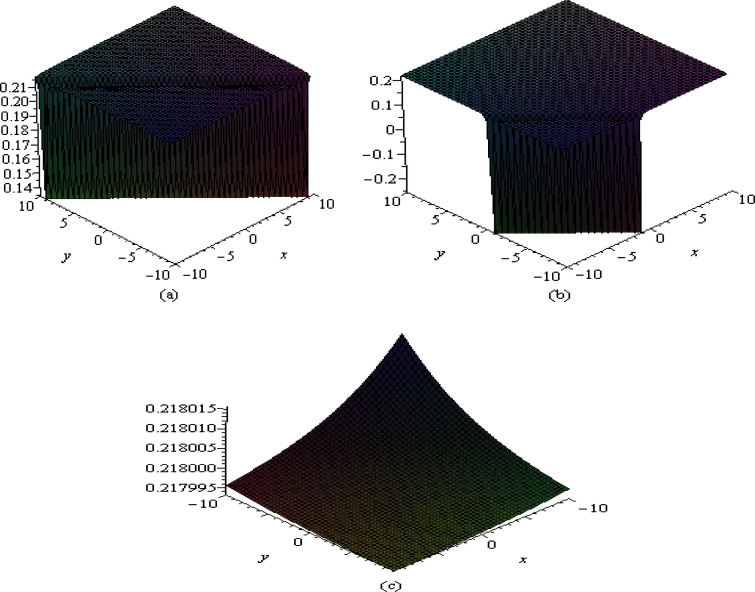Figure 6. Shape of ${\textstyle v_{3}}$ in ${\textstyle x}$–${\textstyle y}$ plane at (a) ${\textstyle t=0}$, (b) ${\textstyle t=10}$ and (c) ${\textstyle t=30}$.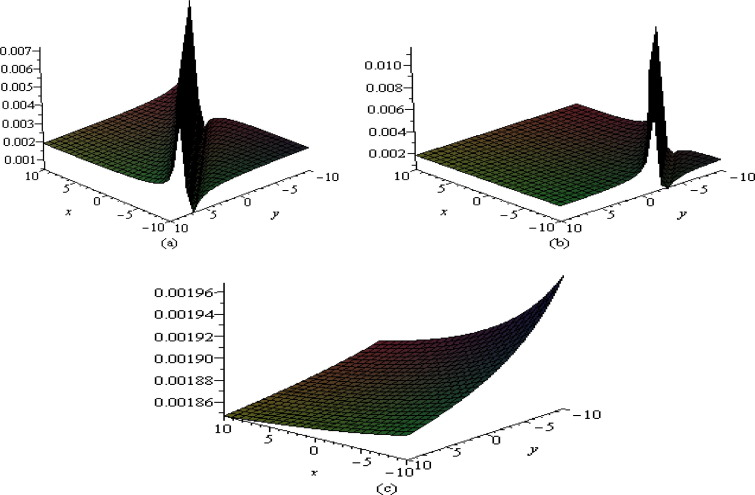Figure 7. Shape of ${\textstyle \vert u_{4}\vert }$ in ${\textstyle x}$–${\textstyle y}$ plane at (a) ${\textstyle t=0}$, (b) ${\textstyle t=10}$ and (c) ${\textstyle t=30}$.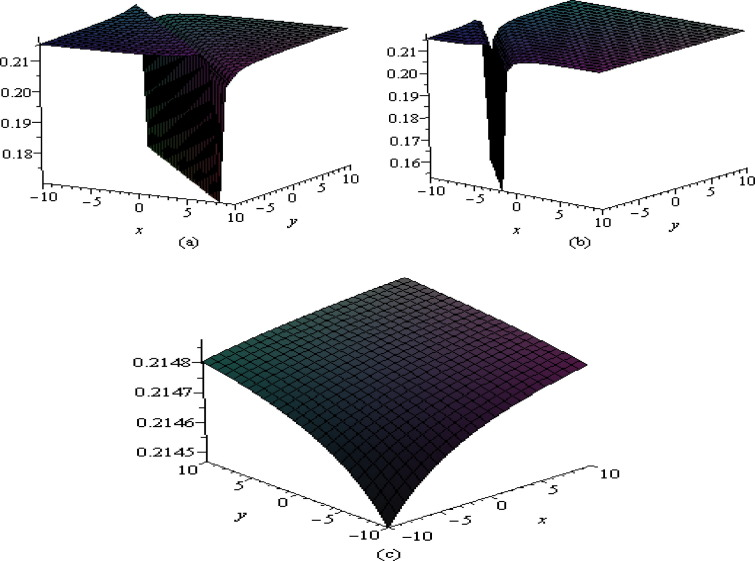Figure 8. Shape of ${\textstyle v_{4}}$ in ${\textstyle x}$–${\textstyle y}$ plane at (a) ${\textstyle t=0}$, (b) ${\textstyle t=10}$ and (c) ${\textstyle t=30}$.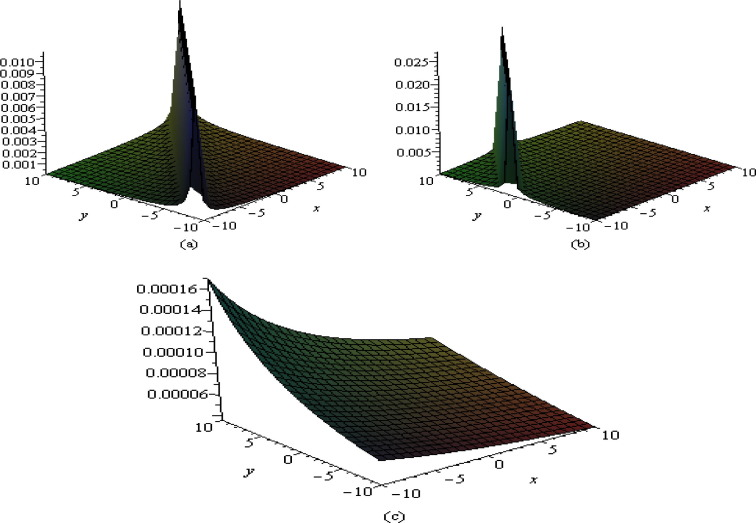Figure 9. Shape of ${\textstyle \vert u_{5}\vert }$ in ${\textstyle x}$–${\textstyle y}$ plane at (a) ${\textstyle t=0}$, (b) ${\textstyle t=10}$ and (c) ${\textstyle t=30}$.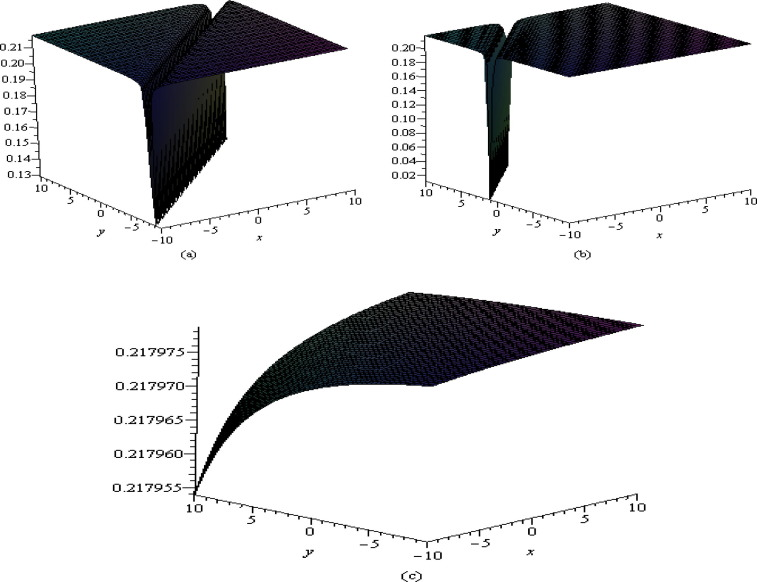Figure 10. Shape of ${\textstyle v_{5}}$ in ${\textstyle x}$–${\textstyle y}$ plane at (a) ${\textstyle t=0}$, (b) ${\textstyle t=10}$ and (c) ${\textstyle t=30}$.

The shape of the solutions ${\textstyle u_{1}}$ and ${\textstyle v_{1}}$ of the coupled Klein–Gordon–Zakharov equation is presented in Figure 1 and Figure 2 respectively with some fixed parametric values ${\textstyle k_{1}=2}$, ${\textstyle k_{2}=1}$, ${\textstyle k_{3}=2}$, ${\textstyle p=1}$, ${\textstyle q=-1}$, ${\textstyle r=1}$, ${\textstyle c_{0}=0.1}$, ${\textstyle E=1}$, ${\textstyle \lambda =8}$, ${\textstyle \mu =2}$, ${\textstyle f_{0}=1}$, ${\textstyle \Omega =5}$, ${\textstyle \delta =5}$, and ${\textstyle \beta =3}$ at times ${\textstyle t=0}$, 10, 25. From Figure 1 and Figure 2, it is observed that the traveling wave solutions ${\textstyle u_{1}}$ and ${\textstyle v_{1}}$ initially form kink type soliton in the ${\textstyle x}$${\textstyle y}$ plane. Kink waves are special type of traveling waves which come up from one asymptotic state to another. It is also observed that the amplitude and shape of these waves become smaller and smaller after interactions. At the final state, they reduce to zero, which shows that the solitonic excitations are not completely elastic.

Figure 3 and Figure 4 show the wave profiles corresponding to the solutions ${\textstyle u_{2}}$ and ${\textstyle v_{2}}$ considering the fixed values of the parameters ${\textstyle k_{1}=2}$, ${\textstyle k_{2}=-1}$, ${\textstyle k_{3}=2}$, ${\textstyle p=1}$, ${\textstyle q=1}$, ${\textstyle r=1}$, ${\textstyle c_{0}=0.1}$, ${\textstyle E=1}$, ${\textstyle \lambda =4}$, ${\textstyle \mu =6}$, ${\textstyle f_{0}=1}$, ${\textstyle \Omega =0.5}$, ${\textstyle \delta =5}$, and ${\textstyle \beta =3}$ for different values of ${\textstyle t=0}$, 10, 1000. The exact solutions ${\textstyle u_{2}}$ and ${\textstyle v_{2}}$ of Eq. (11) are represented periodic wave solutions in the ${\textstyle x}$${\textstyle y}$ plane for different values of t. It is shown that the periodic wave solutions remain unchanged. That is, the periodic wave solution is completely elastic solution after nonlinear interaction.

Figure 5 and Figure 6 present the wave profiles corresponding to ${\textstyle u_{3}}$ and ${\textstyle v_{3}}$ with fixed parametric values ${\textstyle k_{1}=2}$, ${\textstyle k_{2}=-1}$, ${\textstyle k_{3}=2}$, ${\textstyle p=1}$, ${\textstyle q=1}$, ${\textstyle r=1}$, ${\textstyle c_{0}=0.1}$, ${\textstyle E=1}$, ${\textstyle \lambda =0.1}$, ${\textstyle \mu =0}$, ${\textstyle f_{0}=1}$, ${\textstyle \Omega =5}$, ${\textstyle \delta =5}$, and ${\textstyle \beta =3}$ for ${\textstyle t=0}$, 10, 30. The exact solutions ${\textstyle u_{3}}$ and ${\textstyle v_{3}}$ of Eq. (11) initially formed bell type solitary wave in the ${\textstyle x}$${\textstyle y}$ plane. The amplitude and width of these waves also become smaller and smaller after interaction according to increase of time t. It is investigated that the shapes of the bell type solitary wave are changed, but the wave propagation of plasma does not reduce to zero.

Figure 7 and Figure 8 exhibit the wave profiles of ${\textstyle u_{4}}$ and ${\textstyle v_{4}}$ with fixed parametric values ${\textstyle k_{1}=2}$, ${\textstyle k_{2}=-1}$, ${\textstyle k_{3}=2}$, ${\textstyle p=1}$, ${\textstyle q=1}$, ${\textstyle r=1}$, ${\textstyle c_{0}=0.1}$, ${\textstyle E=1}$, ${\textstyle \lambda =1}$, ${\textstyle \mu =2}$, ${\textstyle f_{0}=1}$, ${\textstyle \Omega =5}$, ${\textstyle \delta =5{\mbox{,}}}$ and ${\textstyle \beta =3}$ at times ${\textstyle t=0}$, 10, 30. The exact solutions ${\textstyle u_{4}}$ and ${\textstyle v_{4}}$ of Eq. (11) initially represented singular kink traveling wave solutions in the ${\textstyle x}$${\textstyle y}$ plane.

Figure 9 and Figure 10 present the wave profiles of the solutions ${\textstyle u_{5}}$ and ${\textstyle v_{5}}$ taking the fixed values of the parameters ${\textstyle k_{1}=2}$, ${\textstyle k_{2}=-1}$, ${\textstyle k_{3}=2}$, ${\textstyle p=1}$, ${\textstyle q=-1}$, ${\textstyle r=1}$, ${\textstyle c_{0}=0.1}$, ${\textstyle E=1}$, ${\textstyle \lambda =0}$, ${\textstyle \mu =0}$, ${\textstyle f_{0}=1}$, ${\textstyle \Omega =5}$, ${\textstyle \delta =5}$, and ${\textstyle \beta =3}$ for ${\textstyle t=0}$, 10, 1000. The exact solutions ${\textstyle u_{5}}$ and ${\textstyle v_{5}}$ of Eq. (11) are initially represented cupson and soliton wave propagation respectively in the ${\textstyle x}$${\textstyle y}$ plane. Cupson is a special type of soliton wave propagation.

## 5. Conclusion

The modified ${\textstyle exp(-\phi (\xi ))}$-expansion method has been implemented to construct the new explicit solutions of the coupled (3+1) dimensional Klein–Gordon-Zakharov equation. The hyperbolic, trigonometric, exponential and rational function type traveling wave solutions are obtained considering the fixed values of the related parameters. The annihilation phenomena of the wave propagation in the ${\textstyle x}$${\textstyle y}$ plane are also described. It is observed that the soliton solutions of kink type are not completely elastic, but the periodic traveling wave solutions are completely elastic. It can be concluded that the method presented here is very effective to solve many other nonlinear evolution equations. In future, this method may be applied to find the analytical solutions of the (3+1)-dimensional KdVB equation for investigating the oblique propagation of wave dynamics in plasma physics. Those results will be reported in future publications.

### Document informationPublished on 12/04/17

Licence: Other

### Document Score0

Views 75
Recommendations 0